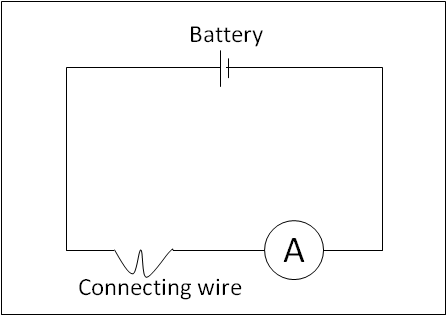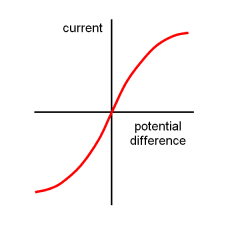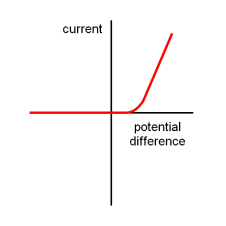# Current Voltage Characteristics

## Introduction

In this article, the relationship between current and voltage for an ohmic conductor, semiconductor diode and filament lamp is discussed. Ohm’s law is also discussed.

If the relationship between the current through the conductor and the potential difference across its end is explored, the relationship will vary according to the circuit element. Conductors, for which the current-voltage characteristics are a straight line, are said to be ohmic conductors. A metal at constant temperature exhibits this kind of relationship. When the current-voltage characteristics exhibit a non-linear behaviour, the conductor is said to be non-ohmic. A lamp filament and a semiconductor diode are two examples of non-ohmic conductors.

A. Ohm’s Law for Ohmic Conductors

Let us find out the relationship between current and voltage of an ohmic conductor by using a circuit as shown in Figure 1. If we connect a battery to the length of the connecting wire, the current through the connecting wire can be measured using an ammeter. The cells in the battery are changed to 1, 2, 3 or 4 cells and the respective current is noted in a table as
shown below.Figure 1: Circuit to Find the Resistance of a Connecting WireThe results obtained are plotted in a graph as shown in Figure 2. The result shows that there is a simple relationship between the potential difference across the connecting wire and the current through it. When this experiment is repeated for other wires, similar relationships are obtained. The graph is always a straight line passing through the origin. This relationship was first discovered by the German physicist Georg Ohm in 1826 and is called as Ohm’s law.Figure 2: Current Versus Potential Difference of an Ohmic Conductor

Provided the temperature and the other physical factors remain constant, the current through a wire is proportional to the potential difference across its ends. When a current of 1 A passes through a conductor of 1Ω, it has a potential difference of 1 V across it. This results in a simple mathematical relationship, which states Ohm’s law.B. Filament Lamp

A filament lamp is made of a thin metallic wire. Let us consider a similar circuit as shown in Figure 1 but now the connecting wire is replaced with a filament lamp to find its current-voltage characteristics. The results obtained are shown in Figure 3. At first, the graph shows a straight line relationship. Hence, the resistance is constant in this region. Then, the graph curves, which means that the resistance increases with current in this region.

The current-voltage characteristics of a filament lamp and a metallic wire are different. There is one more important difference related to the temperature. The temperature of a metallic wire is constant in this experiment. But for a filament lamp, the temperature increases to about 1500°C. The temperature of a filament does not remain constant. The temperature of the filament increases with the current flowing through it. Hence, it does not obey
Ohm’s law.Figure 3: Current-voltage Characteristics of a Filament Lamp

C. Diode

A diode is made of semiconducting material. The circuit symbol for a diode is shown in Figure 4. The diode conducts when the current is in the direction of the arrowhead symbol. This condition is called forward bias. In this case, the potential on the left hand side of the diode is more positive than the potential on the right hand side. When the voltage is reversed, the diode is said to be in reverse bias. The diode does not conduct in reverse bias.Figure 4: Circuit Symbol of Diode

Diodes are non-ohmic conductors. The resistance of the diode is very high for low voltages in the forward bias, i.e. for voltage less than built-in voltage of the diode. The built-in voltage varies for diodes made of different materials. For example: a silicon diode has a built-in voltage of 0.7 V and Germanium diodes have a built-in voltage of 0.3 V. For voltages above this value, the resistance of the diode decreases in forward bias. The current voltage characteristics of a diode is shown in Figure 5 below.Figure 5: Current-voltage Characteristics of a Diode

D. Summary

• Ohm’s law: For a conductor at constant temperature, the current in the conductor is proportional to the potential difference across it.
• The resistance of a metallic wire increases with increasing temperature.
• The temperature of a filament lamp increases with the current flowing through it. It is a non-ohmic conductor.
• The resistance of a semiconductor decreases with increasing temperature.
• A diode has a low resistance when connected in forward bias, and a very high resistance when connected in reverse bias, hence a non-ohmic conductor.